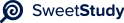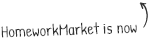•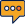0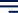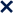1 / 15100%MAT 230 EXAM TWO
This document is proprietary to Southern New Hampshire University. It and the problems within
may not be posted on any non-SNHU website.
Jacqueline Amoah
1|
| | |
| |
| |
| |
Directions: Type your solutions into this document and be sure to show all steps for arriving at
your solution. Just giving a final number may not receive full credit.
P
ROBLEM
1
This question has 2 parts.
Part 1:
Suppose that F and X are events from a common sample space with P (F ) 0 and P (X) 0.
(a)
Pro
v
e
that
P
(
X
)
=
P
(
X
F
)
P
(
F
)
+
P
(
X
F
¯
)
P
(
F
¯
).
Hin
t:
Explain
wh
y
P
(
X
F
)
P
(
F
)
=
P (X F ) is another way of writing the definition of conditional probability, and then use
that with the logic from the proof of Theorem 4.1.1.
P (X F )P (F ) = P (X F ) is
i
true
i
because
i
we
i
want
i
to
i
get
i
all
i
probabilities
i
of
i
both
i
events
i
occurring
i
but
i
want
i
to
i
avoid
i
counting
i
those
i
events
i
that
i
have
i
i
been
i
counted.
i
When
i
the
i
intersection
i
of
i
the
i
probabilities
i
is
i
subtracted
i
we
i
take
i
away
i
those
i
that
i
have
i
been
i
counted
i
twice.
Since
i
all
i
probabilities
i
must
i
i
up
i
to
i
1
i
and
i
no
i
more
i
we
i
can
i
say
i
that
i
the
i
i
of
i
the
i
original
i
probabilities
i
and
i
the
i
complements
i
of
i
said
i
probabilities
i
will
i
give
i
a
i
value
i
of
i
1.
(b)
Explain why P (F X) = P (X F )P (F )/P (X) is another way of stating Theorem 4.2.1 Bayes’
Theorem.
since
P(x)=
P
(
X
F
)
P
(
F
)
+
P
(
X
F
¯
)
P
(
F
¯
)
and bayes theorem is as follows:
p
(
X
|
F
)
p
(
F
)
¯
¯
p
(
X
|
F
)
p
(
F
)+
p
(
X
|
F
)
p
(
F
)
we
i
can
i
see
i
that
i
the
i
denominator
i
and
i
P(x)
i
are
i
equal
i
so
i
can
i
substitute.
Part 2:
A website reports that 70% of its users are from outside a certain country. Out of their users
from outside the country, 60% of them log on every day. Out of their users from inside the country,
80% of them log on every day.
(a)
What percent of all users log on every day? Hint: Use the equation from Part 1 (a).
O= OUTSIDE
I=INSIDE
E=EVERYDAY
p(O)=0.7
p(I)=0.8
p(E)=0.6
P(x)=
P
(
X
F
)
P
(
F
)
+
P
(
X
F
¯
)
P
(
F
¯
)
P(X)= (1-.8)(0.6)+(0.7)(0.8)=0.68
The probability is.68 or 68 percent.
(b)
Using Bayes Theorem, out of users who log on every day, what is the probability that they
are from inside the country?Baye’s Theorem:
p
(
X
|
F
)
p
(
F
)
¯
¯
p
(
X
|
F
)
p
(
F
)+
p
(
X
|
F
)
p
(
F
)
= (0.30)(0.8)/((0.3*.6)+(0.7*.4))=0.521
The probability is 0.521 or 52.1 percent.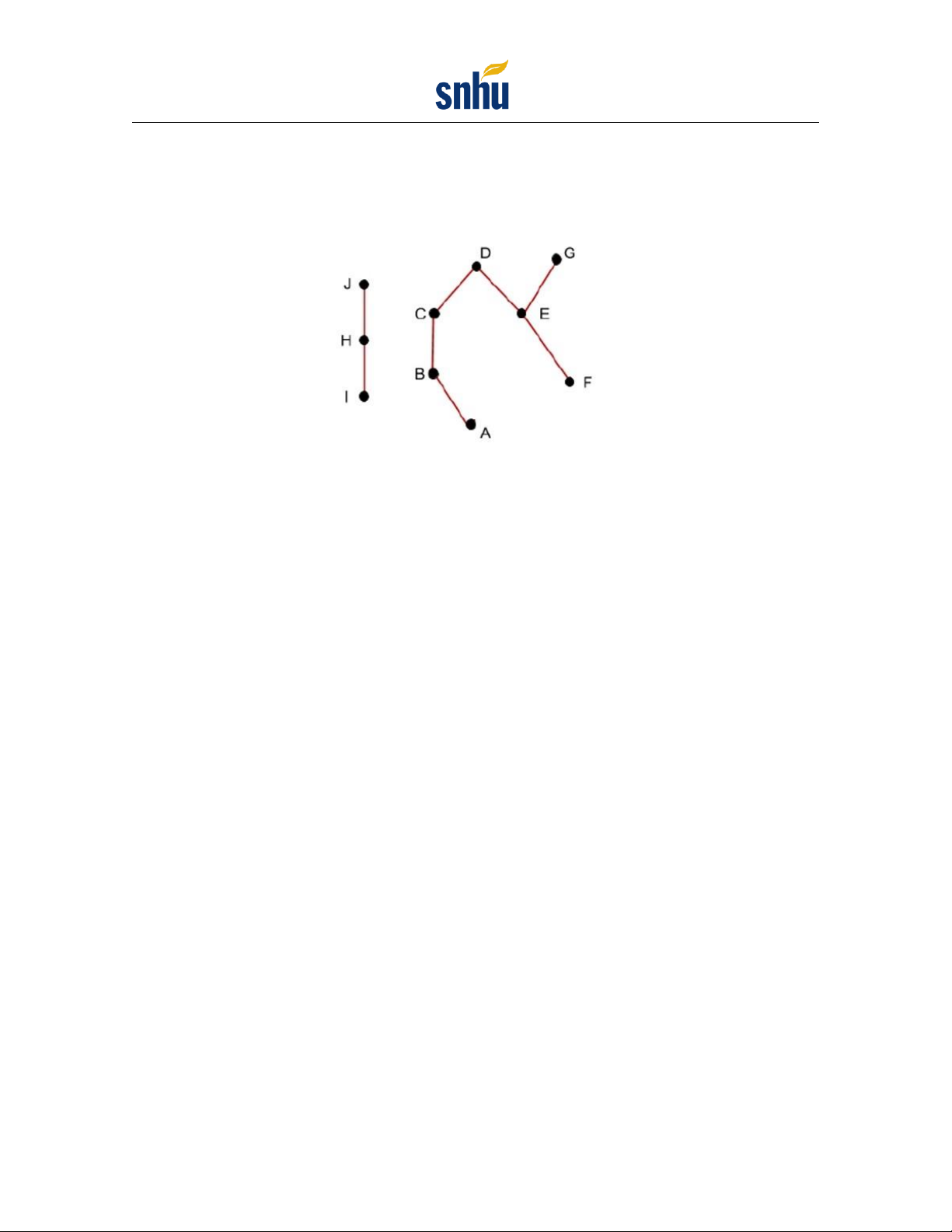/
/
P
ROBLEM
2
This question has 2 parts.
Part 1:
The drawing below shows a Hasse diagram for a partial order on the set:
{
A, B, C, D, E, F, G, H, I,
J
}
Figure 1:
A Hasse diagram shows 10 vertices and 8 edges. The vertices, represented by dots, are as
follows: vertex J is upward of vertex H; vertex H is upward of vertex I; vertex B is inclined upward to
the left of vertex A; vertex C is upward of vertex B; vertex D is inclined upward to the right of vertex
C; vertex E is inclined upward to the left of vertex F; vertex G is inclined upward to the right of vertex
E. The edges, represented by line segments between the vertices are as follows: 3 vertical edges connect
the following vertices: B and C, H and I, and H and J; 5 inclined edges connect the following vertices:
A and B, C and D, D and E, E and F, and E and G.
Determine the properties of the Hasse diagram based on the following questions:
(a)
What are the minimal elements of the partial order?
Minimal elements hold
y=
x and y x.
On
i
the
i
diagram
i
it
i
would
i
be
i
the
i
points
i
with
i
no
i
connections
i
below
i
so,
i
I,A,F
i
are
i
the
i
minimal
i
elements.
(b)
What are the maximal elements of the partial order?
Maximal elements hold: y x an y x.
On
i
the
i
diagram
i
it
i
is
i
the
i
points
i
with
i
no
i
connections
i
above
i
them
i
so,
i
J,D,G
i
are
i
the
i
maximal
i
elements.
(c)
Which of the following pairs are comparable?
(A, D), (J, F ), (B, E), (G, F ), (D, B), (C, F ), (H, I), (C, E)
Comparable
i
ordered
i
pairs
i
are
i
pairs
i
that
i
can
i
be
i
reached
i
by
i
travelling
i
in
i
a
i
single
i
direction.
i
Out
i
of
i
the
i
list
i
the
i
following
i
are
i
comparable:
(A,D),(D,B),(G,F),(H,I)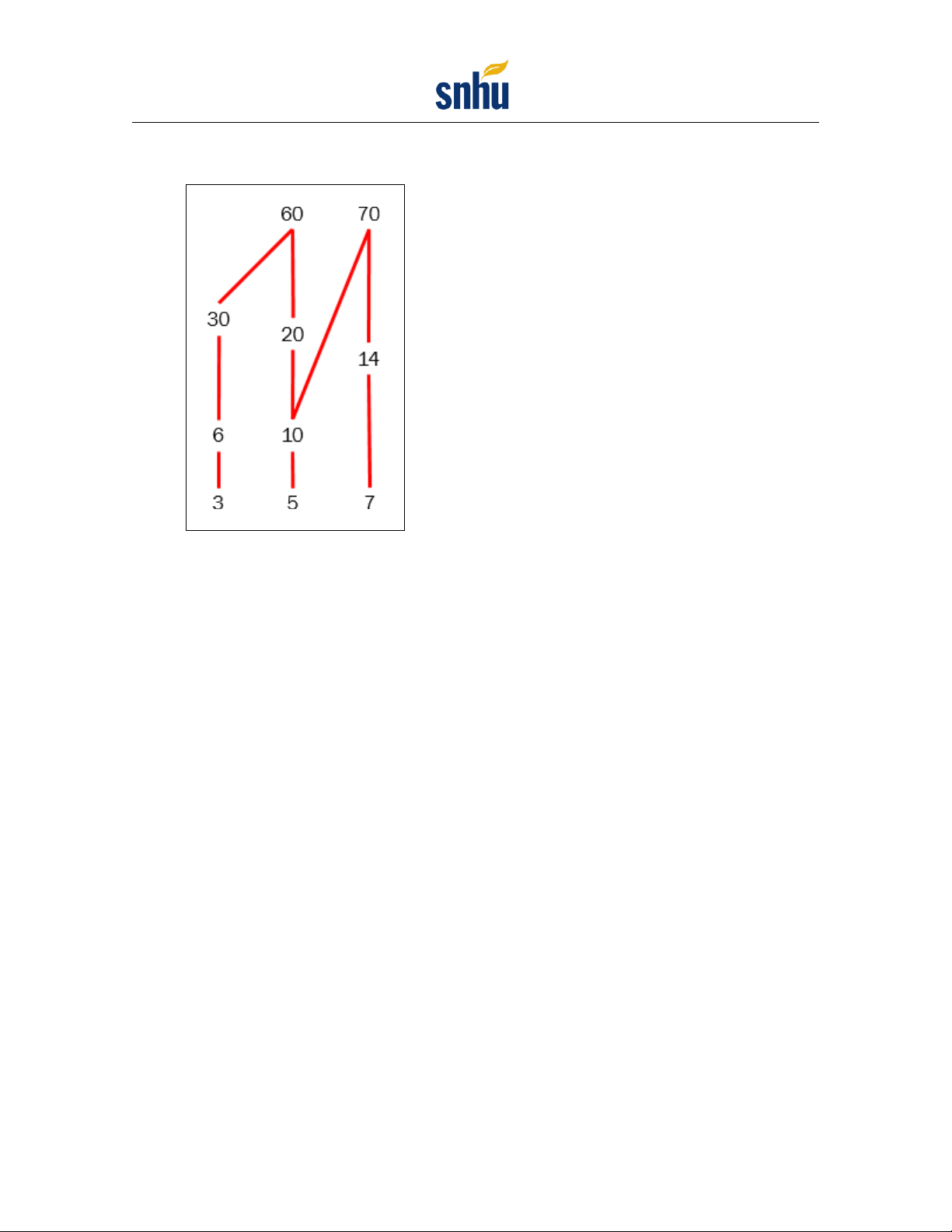Part 2:
Consider the partial order with domain 3, 5, 6, 7, 10, 14, 20, 30, 60, 70 and with x y if
x evenly divides y. Select the correct Hasse diagram for the partial order.
(a)
Figure 2:
A Hasse diagram shows a set of elements 3; 5; 6; 7; 10; 14; 20; 30; 60, 70.
There are lines connecting 3 and 6, 6 and 30, 30 and 60, 5 and 10, 10 and 20, 20 and 60, 10
and 70, 7 and 14, 14 and 70.
This diagram is incorrect because the 10 is not connected to the 30 or the 60. Also the 5
is not connected to its multiple of 30.